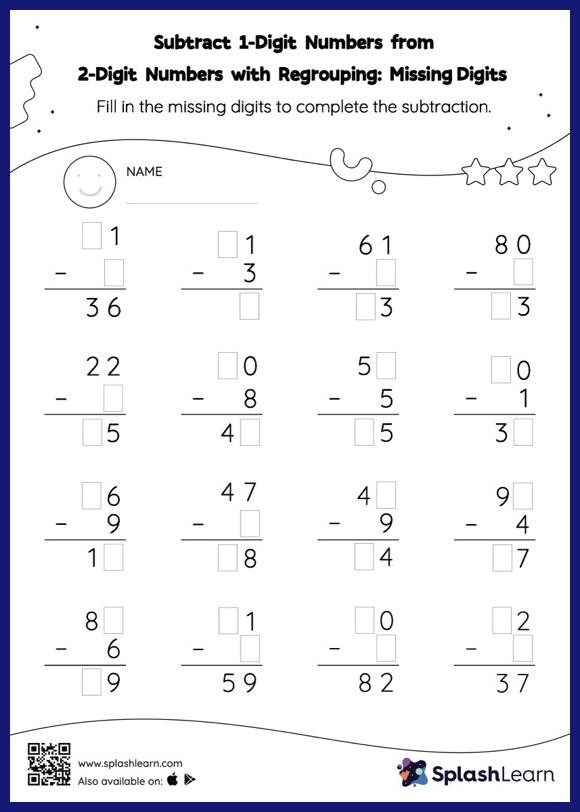# Subtract 1-Digit Numbers from 2-Digit Numbers with Regrouping: Missing Digits Worksheet

Home > Subtract 1-Digit Numbers from 2-Digit Numbers with Regrouping: Missing DigitsStudents use the count back strategy or the relationship between addition and subtraction to find the missing number in this worksheet. They also need to regroup numbers to get to the answer in subtract 1-digit numbers from 2-digit numbers with regrouping worksheet. In each problem, the numbers are laid out in the vertical format. In this format, pair of digits in each successive place are tackled one by one. This helps students follow a structured approach.